# Chemical Kinetics Notes for Class 12 Chemistry

0
1388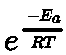CBSE Class 12 Chemistry
Quick Revision Notes
Chapter 4
Chemical Kinetics

Where d[B] is small change in conc. of ‘B’ and dt is small interval of time

Chemical kinetics: It is the branch of chemistry that deals with the study of reaction rates and their mechanisms.

Rate of reaction: It is the change in concentration of reactant (or product) in unit time.

The unit of rate of reaction is mol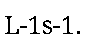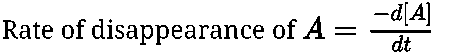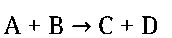where d[A] is small change in conc. of ‘A’ and dt is small interval of time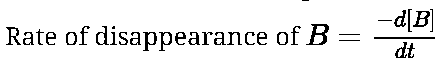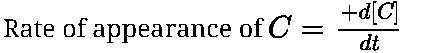Where d[C] is small change in conc. of ‘C’ and dt is small interval of time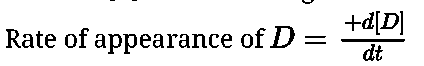Where d[D] is small change in conc. of ‘D’ and dt is small interval of time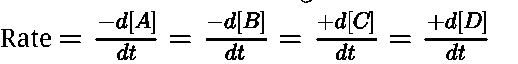Rate law or rate equation: It is the expression which relates the rate of reaction with concentration of the reactants. The constant of proportionality ‘k’ is known as rate constant.

Average rate: It is the rate of reaction measured over a long time interval.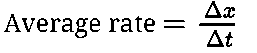where is Ax change in concentration and At is large interval of time.

Instantaneous rate: It is the rate of reaction when the average rate is taken over a particular moment of time. Instantaneous rate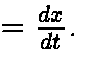where dx is small change in conc. and dt is the smallest interval of time.

It is the expression which relates the rate of reaction with concentration of the reactants.

Rate constant: When the concentration of reactants is unity, then the rate of reaction

is known as rate constant. It is also called specific reaction rate.

The constant of proportionality ‘k’ is known as rate constant.

Molecularity of a reaction: The total number of atoms, ions or molecules of the reactants involved in the reaction is termed as its molecularity. It is always in whole number and is never more than three. It cannot be zero.

Order of a reaction: The sum of the exponents (power) of the concentration of reactants in the rate law is termed as order of the reaction. It can be in fraction. It can be zero also.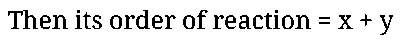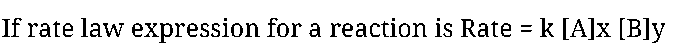Order cannot be determined with a given balanced chemical equation. It can be experimentally determined.

Integrated rate law for zero order reaction: R ^ P

If we plot a graph between concentration of R vs time t, the graph is a straight line with slope equal to -k and intercept is equal to [Ro].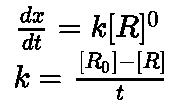• Half- life of a reaction: The time taken for a reaction, when half of the starting material has reacted is called half- life of a reaction.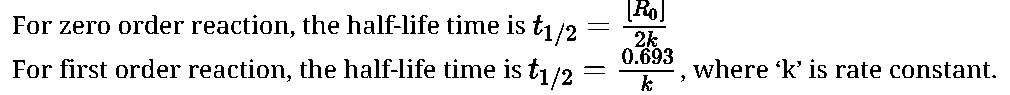It is independent of initial concentration for first order reaction.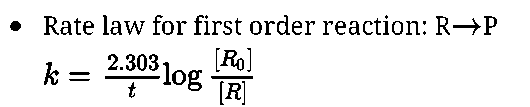where ‘k’ is rate constant or specific reaction rate, [Ro] is initial molar conc., [R] is final molar conc. after time ‘t’.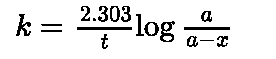where ‘a’ is initial conc. reacted in time ‘t’ final conc., after time ‘t’ is (a – x).

• If we plot a graph between ln[R] with time, we get a straight line whose slope = – k and intercept ln[Ro].
• To calculate rate constant for first order gas phase reaction of the type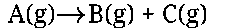Where pi is initial pressure of A, pt is total pressure of gaseous mixture containing A , B, C

• Pseudo first order reaction: The reaction which is bimolecular but order is one is called pseudo first order reaction. This happens when one of the reactants is in large excess.Example – Acidic hydrolysis of ester (ethyl acetate).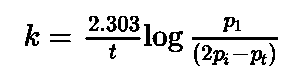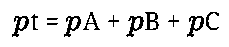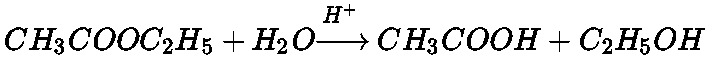• Activation energy (Ea): It is extra energy which must be possessed by reactant molecules so that collision between reactant molecules is effective and leads to the formation of product molecules.
• Arrhenius equation of reaction rate: It gives the relation between rate of reaction and temperature.

where k = rate constant, A = frequency factor, Ea = energy of activation R = gas constant, T = temperature in Kelvin,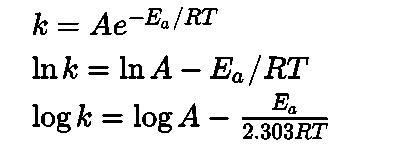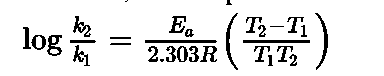• Probability factor or Steric factor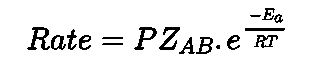Where ZAB represents the collision frequency of reactants, A and B,represents the fraction of molecules with energies equal to or greater than Ea and P is called the probability or steric factor.• Mechanism of reaction: It is the sequence of elementary processes leading to the overall stoichiometry of a chemical reaction.
• Activated complex: It is an unstable intermediate formed between reacting molecules. Since, it is highly unstable and it readily changes into product.
• Rate determining step: It is the slowest step in the reaction mechanism.
• The number of collisions per second per unit volume of the reaction mixture is known as collision frequency (Z).
Previous articleElectro chemistry Notes for Class 12 Chemistry
Next articleSurface Chemistry Notes for Class 12 Chemistry